# 8.e. Simplifying the Solution

After every turn, the length of the recorded path increases by 1. If your maze, for example, has a long zigzag passageway with no side exits, you’ll see a sequence like ‘RLRLRLRL’ appear on the 3pi’s LCD. There’s no shortcut that would get you through this section of the path faster than just following the left hand on the wall strategy. However, whenever we encounter a dead end, we can simplify the path to something shorter.

Consider the sequence ‘LBL’, where ‘B’ stands for “back” and is the action taken when a dead end is encountered. This is what happens if there is a left turn that branches off of a straight path and leads immediately to a dead end. After turning 90° left, 180°, and 90° left again, the net effect is that the robot is heading in its original direction again. The path can be simplified to a 0° turn: a single ‘S’. The following diagram depicts this scenario, showing the two functionally equivalent paths from start to end: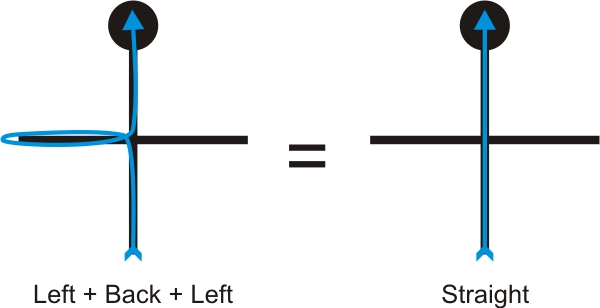Another example is a T-intersection with a dead end on the left: ‘LBS’. The turns are 90° left, 180°, and 0°, for a total of 90° right. The sequence should be replaced with a single ‘R’.

In fact, whenever we have a sequence like ‘xBx’, we can replace all three turns with a turn corresponding to the total angle, eliminating the U-turn and speeding up our solution. Here’s the code to handle this:

// Path simplification.  The strategy is that whenever we encounter a
// sequence xBx, we can simplify it by cutting out the dead end.  For
// example, LBL -> S, because a single S bypasses the dead end
// represented by LBL.
void simplify_path()
{
// only simplify the path if the second-to-last turn was a 'B'
if(path_length < 3 || path[path_length-2] != 'B')
return;

int total_angle = 0;
int i;
for(i=1;i<=3;i++)
{
switch(path[path_length-i])
{
case 'R':
total_angle += 90;
break;
case 'L':
total_angle += 270;
break;
case 'B':
total_angle += 180;
break;
}
}

// Get the angle as a number between 0 and 360 degrees.
total_angle = total_angle % 360;

// Replace all of those turns with a single one.
switch(total_angle)
{
case 0:
path[path_length - 3] = 'S';
break;
case 90:
path[path_length - 3] = 'R';
break;
case 180:
path[path_length - 3] = 'B';
break;
case 270:
path[path_length - 3] = 'L';
break;
}

// The path is now two steps shorter.
path_length -= 2;
}

One interesting point about this code is that there are some sequences that should never be encountered by a left-turning robot, like ‘RBR’, which would be replaced by ‘S’ according to this code. In a more advanced program, you might want to keep track of inconsistencies like this, since they indicate some kind of a problem that could cause the robot to get lost.

Now let’s step through a slightly more complicated maze, showing how we can simplify the path as we explore it:

Fully explore the maze using a left-hand-on-the-wall strategy.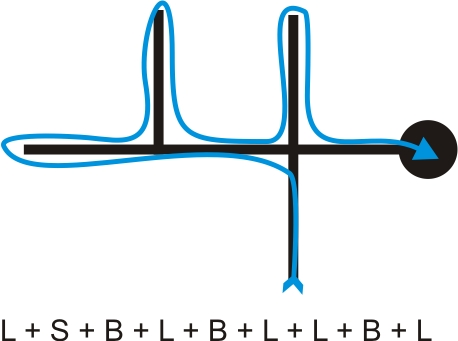The above list of actions is a record of all the steps we took to fully explore the maze while looking for the end, which is marked by the large black circle. Our goal is to now reduce this list to represent the shortest path from start to finish by weeding out all of the dead ends. One option is to perform this pruning when we finish the maze, but the better approach is to perform the pruning as we go to keep our list from growing excessively large and taking up more memory than we have available.

Prune out the first dead end as we identify it.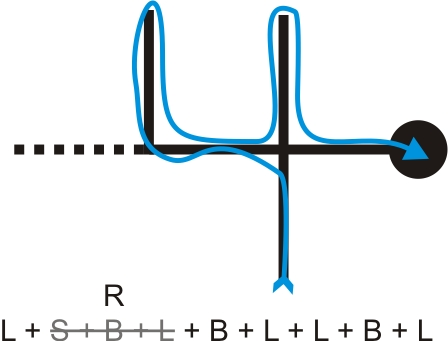When we encounter the first intersection after our first “back” action, we know we have reached a dead end that can be removed from our list of actions. In this case, the most recent actions in our list is the sequence ‘SBL’, and the diagram shows that this sequence can be simplified into a single right turn ‘R’.

Prune out the rest of this dead-end branch as we back-track.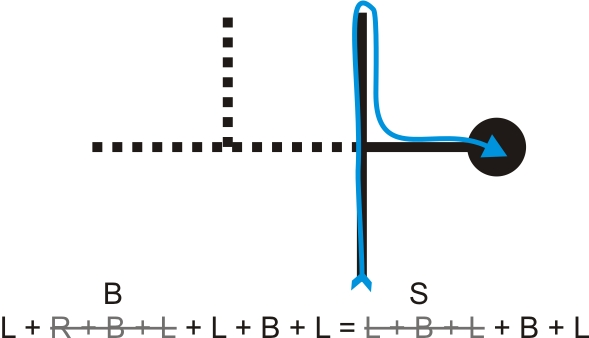We next end up with the sequence ‘RBL’, which reduces to a single back ‘B’, and this combines with the next action to produce the sequence ‘LBL’, which reduces to a single straight ‘S’.

Prune out the final dead-end branch to leave us with the shortest path from start to finish.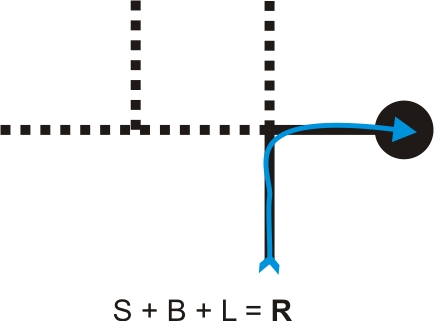The last dead end gives us the sequence ‘SBL’, which reduces to a sigle right turn ‘R’. Our action list is now just ‘R’ and represents the shortest path from start to finish.

As we drove the maze, our action list would have looked like the following:

1. L
2. LS
3. LSB
4. LSBL => LR (pruning occurs here)
5. LRB
6. LRBL => LB (pruning occurs here)
7. LBL => S (pruning occurs here)
8. SB
9. SBL => R (pruning occurs here)